Nonlin. Processes Geophys., 26, 445–456, 2019
https://doi.org/10.5194/npg-26-445-2019
Nonlin. Processes Geophys., 26, 445–456, 2019
https://doi.org/10.5194/npg-26-445-2019

Research article 26 Nov 2019

Research article | 26 Nov 2019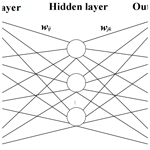# A fast approximation for 1-D inversion of transient electromagnetic data by using a back propagation neural network and improved particle swarm optimization

A fast approximation for 1-D inversion of transient electromagnetic data by using a back propagation neural network and improved particle swarm optimization
Ruiyou Li, Huaiqing Zhang, Nian Yu, Ruiheng Li, and Qiong Zhuang Ruiyou Li et al.
• The State Key Laboratory of Transmission Equipment & System Safety and Electrical New Technology, Chongqing University, Chongqing, 400044, China

Correspondence: Huaiqing Zhang (zhanghuaiqing@cqu.edu.cn)

Abstract

As one of the most active nonlinear inversion methods in transient electromagnetic (TEM) inversion, the back propagation (BP) neural network has high efficiency because the complicated forward model calculation is unnecessary in iteration. The global optimization ability of the particle swarm optimization (PSO) is adopted for amending the BP's sensitivity to its initial parameters, which avoids it falling into a local optimum. A chaotic-oscillation inertia weight PSO (COPSO) is proposed for accelerating convergence. The COPSO-BP algorithm performance is validated by two typical testing functions, two geoelectric models inversions and a field example. The results show that the COPSO-BP method is more accurate, stable and needs relatively less training time. The proposed algorithm has a higher fitting degree for the data inversion, and it is feasible to use it in geophysical inverse applications.

Share
1 Introduction

The transient electromagnetic (TEM) method applies the secondary receiving voltage induced by the rapid switching of pulse current, and it then deduces the geoelectrical parameters consisting of the resistivities and thicknesses of the layers. The later is a typical TEM inversion issue with nonlinear features. The linear inversion method was simple and widely used through linearization processes, yet it is extremely dependent on the selection of initial parameters and results in poor inversion accuracy. Hence, the nonlinear inversion methods have attracted more geophysicists' attention in recent years.

The artificial neural network (ANN) is one of the most active nonlinear inversion methods, and it has very high computation efficiency because the complicated forward model calculation is unnecessary in this iteration. All the geoelectrical parameters and the forward model relations are implied in the weight and threshold parameters of ANN. And it is different from the nonlinear Monte Carlo method with a global space search solution (He et al., 2018; Jha et al., 2008; Pekşen et al., 2014; Sharma, 2012; Tran and Hiltunen, 2012). Srinivas et al. (2012) compared the inversion performance of back propagation (BP), the radial basis function (RBF) and the generalized regression neural network (GRNN) in vertical electrical sounding data, then established a 1-D inversion model with BP and finally realized the parameter inversion. Maiti et al. (2012) proposed a Bayesian neural-network training method in 1-D electrical sounding. Jiang et al. (2018) improved the training method for the kernel principal-component wavelet neural network and achieved the resistivity imaging. Jiang et al. (2016a) produced a learning algorithm based on information criterion (IC) and particle swarm optimization for RBF network which improves the global search ability. Johnson and Aizebeokhai (2017) utilized neural-network method to invert multi-layer georesistivity sounding. Jiang et al. (2016b) presented a pruning Bayesian neural-network (PBNN) method for resistivity imaging and solved the instability and local minimization problems. Raj et al. (2014) solved nonlinear apparent resistivity inversion problems with ANN. The ANN has been widely applied in electric-prospecting data interpretation for its powerful fitting ability. However, the neural-network method is sensitive to its initial parameter settings and falls easily into a local minimum. Many improved methods were proposed for balancing the convergence rate and inversion quality. Zhang and Liu (2011) proposed ant colony optimization for ANN, and they applied high-density resistivity and acquired smaller inversion errors and higher determinant coefficients. Dai et al. (2014) suggested a differential evolution (DE) for BP which enhanced the global search ability. Rosas-Carbajal et al. (2014) introduced the genetic algorithm for ANN.

The particle swarm optimization (PSO) has a simple structure, fast convergence rate, high accuracy and global optimization ability. Fernández et al. (2010) successfully introduced the PSO in a 1-D resistivity inversion. Godio and Santilano (2018) applied it in a geophysical inversion and deduced a depth resistivity earth model. Due to the PSO's global searching performance, the BP's initial weights and thresholds can be trained by the PSO, and the BP's global optimization ability can be improved. Compared to the standard PSO (SPSO), a chaotic-oscillation inertia weight PSO (COPSO) can accelerate the convergence rate in the early stage, and it was proposed naturally (Shi et al., 2009).

The paper structure is as follows: the principles of the PSO algorithm with different inertia weights schemes, the BP neural network and the proposed COPSO-BP algorithm are given in Sect. 2. Then, the COPSO-BP algorithm performance is validated by two typical testing functions in Sect. 3. And in a later section, inversion simulations of three-layer and five-layer geoelectric models are carried out; the hidden-layer neuron numbers determining method is put forward; and algorithm performance is compared.

2 Principle of COPSO-BP algorithms

## 2.1 Chaotic-oscillation PSO algorithm

For an n-dimensional optimization problem, it is supposed that the position (resistivity and thickness for layered-model parameter inversion) and velocity (update speed) of the ith particle (global search group number) at time t are xi= (xi1, xi2, …, xiN) and vi= (vi1, vi2, …, viN), respectively. Then, at time t+1, they can be calculated by the iterations as

$\begin{array}{}\text{(1)}& {v}_{\mathrm{id}}^{t+\mathrm{1}}=\mathit{\omega }\cdot {v}_{\mathrm{id}}^{t}+{c}_{\mathrm{1}}{r}_{\mathrm{1}}\left({p}_{\mathrm{id}}^{t}-{x}_{\mathrm{id}}^{t}\right)+{c}_{\mathrm{2}}{r}_{\mathrm{2}}\left({p}_{\mathrm{gd}}^{t}-{x}_{\mathrm{id}}^{t}\right),\text{(2)}& {x}_{\mathrm{id}}^{t+\mathrm{1}}={x}_{\mathrm{id}}^{t}+{v}_{\mathrm{id}}^{t+\mathrm{1}},\end{array}$

where r1 and r2 are random values evenly distributed in the interval (0,1), c1 and c2 are learning factors (usually equal to 2), and pid and pgd are the individual and global maximum values.

The inertia weight parameter ω affects the algorithm performance seriously. A fixed weight always was used in the early time, and then various dynamic weights were proposed. Shi et al. (2010) have summarized several methods as

$\begin{array}{}\text{(3)}& {\mathit{\omega }}_{\mathrm{1}}\left(t\right)={\mathit{\omega }}_{\mathrm{s}}-\left({\mathit{\omega }}_{\mathrm{s}}-{\mathit{\omega }}_{\mathrm{e}}\right)t/{T}_{max},\text{(4)}& {\mathit{\omega }}_{\mathrm{2}}\left(t\right)={\mathit{\omega }}_{\mathrm{s}}-\left({\mathit{\omega }}_{\mathrm{s}}-{\mathit{\omega }}_{\mathrm{e}}\right)\left(t/{T}_{max}{\right)}^{\mathrm{2}},\text{(5)}& {\mathit{\omega }}_{\mathrm{3}}\left(t\right)={\mathit{\omega }}_{\mathrm{s}}-\left({\mathit{\omega }}_{\mathrm{s}}-{\mathit{\omega }}_{\mathrm{e}}\right)\left[\mathrm{2}t/{T}_{max}-\left(t/{T}_{max}{\right)}^{\mathrm{2}}\right],\end{array}$

where ωs and ωe are the start and end weight. The t and Tmax are the current and maximum iterations. The above weights are smooth and monotonically decreasing. In this paper, we proposed a decreasing oscillation weight scheme which was based on the chaotic logistic equation. Its specific calculation formula is

$\begin{array}{}\text{(6)}& {x}_{t+\mathrm{1}}=\mathit{\mu }{x}_{t}\left(\mathrm{1}-{x}_{t}\right)\phantom{\rule{1em}{0ex}}t=\mathrm{0},\mathrm{1},\mathrm{2},\mathrm{\dots },n,\text{(7)}& {\mathit{\omega }}_{\mathrm{c}}\left(t\right)={\mathit{\omega }}_{\mathrm{e}}+\left({\mathit{\omega }}_{\mathrm{s}}-{\mathit{\omega }}_{\mathrm{e}}\right)\left({\mathrm{0.99}}^{t}\cdot {x}_{t}\right),\end{array}$

where μ is the control parameter. A complete chaos state is established for x(0,1) and μ=4, and an inertia weight is then obtained from Eq. (7). Numerical experiments were carried out correspondingly and showed that the initial value of x0 has little effect on the inertia weight ω. The inertia weight comparison was shown in Fig. 1, where x0=0.234 and μ=4 for chaotic oscillation.

## 2.2 BP neural network

The BP neural network has a multi-layer feed-forward structure, and a typical three-layer network is shown in Fig. 2 (Li et al., 2009).

For Fig. 2, x1,x2, …, xn are the input values, y1, y2, …, ym are the predicted outputs, and wij and wjk are the network weights. The threshold parameter α is defined in the hidden layer with its output,

$\begin{array}{}\text{(8)}& {H}_{j}=f\left(\sum _{i=\mathrm{1}}^{n}{w}_{ij}{x}_{i}-{\mathit{\alpha }}_{j}\right)\phantom{\rule{1em}{0ex}}j=\mathrm{1},\mathrm{2},\phantom{\rule{0.25em}{0ex}}\mathrm{\dots },l,\end{array}$

where l is the hidden-layer node numbers, f is the activation function with different expressions, and the most widely used is a sigmoid-type function. The predicted output for the kth unit is calculated by

$\begin{array}{}\text{(9)}& {O}_{k}=\sum _{j=\mathrm{1}}^{l}{H}_{j}{w}_{jk}-{b}_{k},\end{array}$

and parameter b is the output threshold. Then the prediction error can be determined based on the predicted output Ok and the expected output Tk, which is ${e}_{k}=\left({T}_{k}-{O}_{k}\right){O}_{k}\left(\mathrm{1}-{O}_{k}\right)$. The updated formula for weights and thresholds is the following:

$\begin{array}{}\text{(10)}& \left\{\begin{array}{c}{w}_{ij}={w}_{ij}+\mathit{\eta }{H}_{j}\left(\mathrm{1}-{H}_{j}\right){x}_{i}{\sum }_{k=\mathrm{1}}^{m}{w}_{jk}{e}_{k}\\ {w}_{jk}={w}_{jk}+\mathit{\eta }{H}_{j}{e}_{k}\\ {\mathit{\alpha }}_{j}={\mathit{\alpha }}_{j}+\mathit{\eta }{H}_{j}\left(\mathrm{1}-{H}_{j}\right){\sum }_{k=\mathrm{1}}^{m}{w}_{jk}{e}_{k}\\ {b}_{k}={b}_{k}+{e}_{k}\end{array},\right\\end{array}$

where i is 1,2, …, n, j is 1,2, …, l, k is 1,2, …, m and η is the learning rate.

## 2.3 BP neural network with the COPSO algorithm

The initial parameters are chosen randomly, which affects the convergence rate, learning efficiency and perhaps falling into a local minimum. The chaotic-oscillation PSO (COPSO) has a much better global optimization capability; therefore, the COPSO algorithm is proposed to optimize the initial weight and threshold of the BP. The COPSO-BP pseudo-codes are briefly described in Algorithm 1.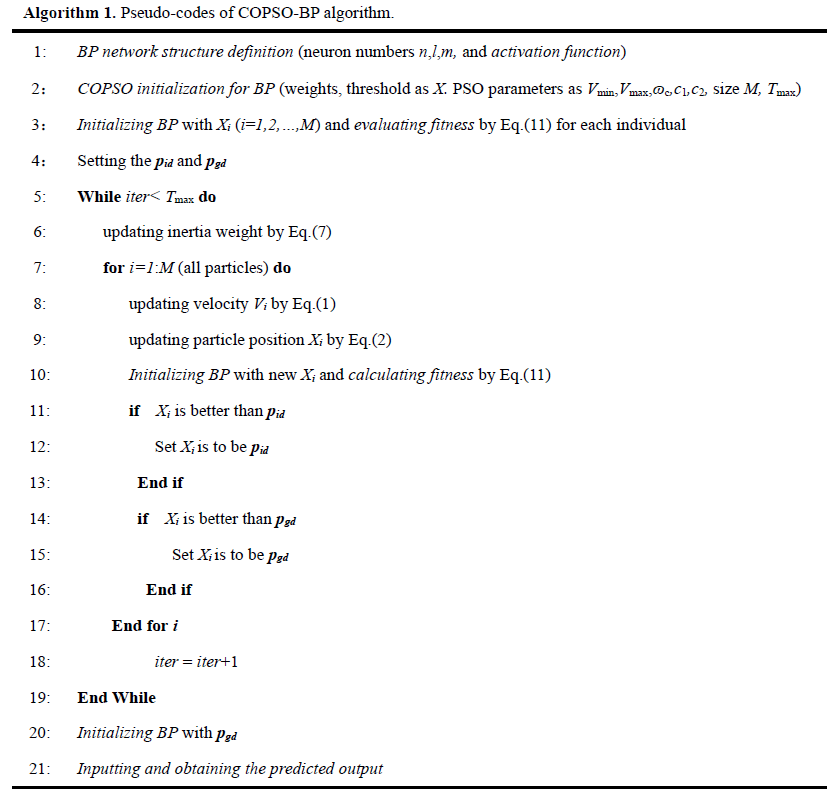The formula for calculating the ith particle fitness is defined as

$\begin{array}{}\text{(11)}& {f}_{i}=\frac{\mathrm{1}}{S}\sum _{s=\mathrm{1}}^{S}\sum _{j=\mathrm{1}}^{m}{\left({Y}_{sj}-{\stackrel{\mathrm{^}}{Y}}_{sj}\right)}^{\mathrm{2}},\end{array}$

where S is the number of training set samples, m is the output neurons number, Ysj is the jth true output of the sth sample, and ${\stackrel{\mathrm{^}}{Y}}_{sj}$ is the corresponding predicted output.

3 Algorithm testing

In order to investigate the COPSO-BP performance and reliability, Rosenbrock and Bohachevsky testing functions were adopted, which are typical non-convex functions and mainly evaluate the performance of unconstrained algorithms. However, due to the random nature of the function, it is not easy to solve and has a global minimum function value of zero.

## 3.1 Rosenbrock function

$\begin{array}{}\text{(12)}& \begin{array}{rl}{f}_{\mathrm{1}}\left(x\right)& =\mathrm{100}×{\left({x}_{\mathrm{1}}^{\mathrm{2}}-{x}_{\mathrm{2}}\right)}^{\mathrm{2}}+{\left(\mathrm{1}-{x}_{\mathrm{1}}\right)}^{\mathrm{2}},{x}_{i}\\ & \in \left[-\mathrm{10},\mathrm{10}\right],i=\mathrm{1},\mathrm{2}\end{array}\end{array}$

## 3.2 Bohachevsky function

$\begin{array}{}\text{(13)}& \begin{array}{rl}{f}_{\mathrm{2}}\left(x\right)& ={x}_{\mathrm{1}}^{\mathrm{2}}+{x}_{\mathrm{2}}^{\mathrm{3}}-{x}_{\mathrm{1}}{x}_{\mathrm{2}}{x}_{\mathrm{3}}+{x}_{\mathrm{3}}-\mathrm{sin}\left({x}_{\mathrm{2}}^{\mathrm{2}}\right)\\ & -\mathrm{cos}\left({x}_{\mathrm{1}}{x}_{\mathrm{3}}^{\mathrm{2}}\right),{x}_{i}\in \left[-\mathrm{2}\mathit{\pi },\mathrm{2}\mathit{\pi }\right],i=\mathrm{1},\mathrm{2},\mathrm{3}\end{array}\end{array}$

Using the standard PSO-BP (SPSO-BP) with linear decreasing inertia weight in Eq. (3), the COPSO-BP tests were carried out. The three-layer BP with an n-s-1 structure is constructed with different hidden nodes. The PSO parameters are the population size M=60, learning factors ${c}_{\mathrm{1}}={c}_{\mathrm{2}}=\mathrm{2.0}$, maximum iteration Tmax=30, inertia weight ωs=0.9, ωe=0.4, x0=0.234 and μ=4 for chaotic parameters, and the search dimension $D=n×s+s×\mathrm{1}+s+\mathrm{1}$, which includes all the neuron weights and thresholds. For BP network, 150 training samples and 50 testing samples were randomly produced within the variable range. The training error is defined as

$\begin{array}{}\text{(14)}& E=\frac{\mathrm{1}}{S}\sum _{s}^{S}{\left({T}_{s}-{O}_{s}\right)}^{\mathrm{2}},\end{array}$

where S is the training samples number and Ts and Os are the expected and predicted outputs for training sample s, respectively. The network structures with minimum training errors for the Rosenbrock and Bohachevsky functions are 2-7-1 and 3-6-1, respectively. The simulation is performed 20 times for each testing function with the SPSO-BP and COPSO-BP algorithms. The numerical result was shown in Table 1. One of the evolutionary training error curves (randomly selected once in 20 times) was shown in Fig. 3, and the fitting curves of the COPSO-BP algorithm were shown in Fig. 4.

Table 1Comparison of the SPSO-BP and COPSO-BP algorithms for testing functions.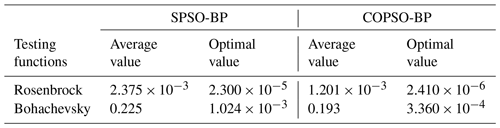It can be seen in Table 1 that although both the SPSO-BP and COPSO-BP algorithms can acquire relatively high accuracy for testing functions, the COPSO-BP algorithm is slightly better than the SPSO-BP algorithm. However, the COPSO-BP algorithm has a better convergence rate and optimization efficiency in the early stage in Fig. 3. Therefore, the SPSO-BP and COPSO-BP algorithms have a strong learning ability, good stability and generalization ability, which will be suitable for TEM inversion.

4 Layered-model and parameter analysis

## 4.1 Forward model

According to the derivation of Kaufman and Keller (1983), the frequency response of the central loop source for the layered model takes the following Hankel transform:

$\begin{array}{}\text{(15)}& {H}_{z}\left(\mathit{\rho },\mathit{\omega }\right)=Ia\underset{\mathrm{0}}{\overset{\mathrm{\infty }}{\int }}\frac{{m}^{\mathrm{2}}}{m+{m}_{\mathrm{1}}/{R}_{\mathrm{1}}^{\ast }}{J}_{\mathrm{1}}\left(m\mathit{\rho }\right)\mathrm{d}m,\end{array}$

where a is the radius of the transmitting coil, I is the excitation current, ρ is the center distance between the transmitting coil and the receiving coil, J1(mρ) is the 1st-order Bessel function, m is the integral variable, m1 is equal to (m2-${k}_{\mathrm{1}}^{\mathrm{2}}{\right)}^{\mathrm{1}/\mathrm{2}}$, k1 is the conduction current, σ1 is the conductivity, k1 is equal to iωμσ1, and ${R}_{\mathrm{1}}^{\ast }$ is the first-layer apparent resistivity conversion function, which can be obtained by the following recurrence formula:

$\begin{array}{}\text{(16)}& \left\{\begin{array}{l}{R}_{n}^{\ast }=\mathrm{1}\\ {R}_{j}^{\ast }=\frac{{m}_{j}{R}_{j+\mathrm{1}}^{\ast }+{m}_{j+\mathrm{1}}\mathrm{th}\left({m}_{j}{h}_{j}\right)}{{m}_{j+\mathrm{1}}+{m}_{j}{R}_{j+\mathrm{1}}^{\ast }\mathrm{th}\left({m}_{j}{h}_{j}\right)}\end{array}.\right\\end{array}$

There is no analytical solution for the time-domain response for the layered model; it can only be solved by numerical calculation. The Hankel transform in formula (15) is calculated by an improved digital filtering algorithm with 47 points, the J1 filter coefficient, and then the time response can be obtained using the Gaver–Stehfest transform as follows:

$\begin{array}{}\text{(17)}& {H}_{z}\left(\mathit{\rho },t\right)=\frac{\mathrm{ln}\mathrm{2}}{t}\sum _{n=\mathrm{1}}^{\mathrm{N}}{K}_{n}{H}_{z}\left(\mathit{\rho },{s}_{n}\right),\end{array}$

where sn is equal to $\left(\mathrm{ln}\mathrm{2}/t\right)×n$, Kn is the coefficient and N is determined by the computer bits, generally N =12.

The ramp excitation current of TEM is

$\begin{array}{}\text{(18)}& I\left(t\right)=\left\{\begin{array}{c}\mathrm{0},t<\mathrm{0}\\ t/{T}_{\mathrm{1}},\mathrm{0}\le t<{T}_{\mathrm{1}}\\ \mathrm{1},{T}_{\mathrm{1}}

where T1 is the turn-off time, and the Laplace transform is

$\begin{array}{}\text{(19)}& I\left(s\right)=\frac{\mathrm{1}}{{T}_{\mathrm{1}}{s}^{\mathrm{2}}}-\frac{\mathrm{1}}{{T}_{\mathrm{1}}{s}^{\mathrm{2}}}{e}^{-{T}_{\mathrm{1}}s}=\frac{\mathrm{1}}{{T}_{\mathrm{1}}{s}^{\mathrm{2}}}\left(\mathrm{1}-{e}^{-{T}_{\mathrm{1}}s}\right).\end{array}$

Therefore, for a specific layered model, the apparent resistivity conversion function ${R}_{\mathrm{1}}^{\ast }$ is firstly calculated by the recurrence in Eq. (16) based on geoelectric structure parameters. And then the frequency response at fixed point Hz(ω) is calculated by a Hankel transform as in Eq. (15). For ramp excitation, the Laplace transform of Hz(s) should multiplied by I(s). Finally, the time response Hz(t) is obtained by a Gaver–Stehfest transform in Eq. (17). So the Hz(t) is obtained by a Gaver–Stehfest transform, a Hankel transform and a recurrence calculation, and it is somewhat computationally consuming.

However, the vertical magnetic field Hz(t) is the actual observed signal in the transient electromagnetic method in engineering applications. It is the inversion input, and the outputs are geoelectric structure parameters. A method which can avoid the complicated forward model calculation is of great importance in algorithm efficiency.

## 4.2 BP network design and the COPSO algorithm

For BP structure, the output nodes are determined by the number of inversion geoelectrical parameters, the input nodes are determined by the samples number of Hz(t), and the hidden nodes vary according to approximation performance. As a three-layer or five-layer geoelectric model, its geoelectrical parameters are five (three resistivity and two thickness parameters) or nine (five resistivity and four thickness parameters); the output nodes are five or nine, correspondingly. The characteristic samplings of Hz(t) are chosen as 10 or 20, which are determined by the model's complexity, with more layers meaning more sampling points needed. The 10 samplings were selected in this paper, hence with 10 input nodes. The hidden-layer neuron number is directly related to the weight and threshold parameter amount and greatly affects the BP performance. An appropriate hidden-node number is necessary, and a determination coefficient R2 is defined for evaluation as

$\begin{array}{}\text{(20)}& {R}^{\mathrm{2}}=\frac{{\left(n\sum _{i=\mathrm{1}}^{n}{Y}_{i}{\stackrel{\mathrm{^}}{Y}}_{i}-\sum _{i=\mathrm{1}}^{n}{Y}_{i}\sum _{i=\mathrm{1}}^{n}{\stackrel{\mathrm{^}}{Y}}_{i}\right)}^{\mathrm{2}}}{\left(n\sum _{i=\mathrm{1}}^{n}{\stackrel{\mathrm{^}}{Y}}_{i}^{\mathrm{2}}-{\left(\sum _{i=\mathrm{1}}^{n}{\stackrel{\mathrm{^}}{Y}}_{i}\right)}^{\mathrm{2}}\right)\left(n\sum _{i=\mathrm{1}}^{n}{Y}_{i}^{\mathrm{2}}-{\left(\sum _{i=\mathrm{1}}^{n}{Y}_{i}\right)}^{\mathrm{2}}\right)},\end{array}$

where Yi is the true value, ${\stackrel{\mathrm{^}}{Y}}_{i}$ is the predicted value for the ith training data and n is the training data number. A larger determination coefficient means better approximation performance. The simulations on hidden-nodes effects were carried out for three-layer and five-layer geoelectric models. The BP structure is 10-s-5 and 10-s-9, and its transfer, training and learning functions are the “log sigmoidal”, “Levenberg–Marquardt” and “gradient descent momentum”, respectively. The average, minimum and maximum values of R2 were obtained after running 20 times for each simulation. The R2 curves were shown in Fig. 5.

It can be seen that the optimal neural-network structures were 10-2-5 and 10-5-9 for three- and five-layer models based on the maximum R2 values. Then, the PSO-BP algorithms with different inertia weight were implemented and compared for the three-layer model. The BP structure was chosen as 10-2-5, and four types of inertia weight as in Eqs. (3)–(7) in the PSO were compared in Table 2.

Table 2Comparison of different inertia weights in PSO algorithms (ωs=0.9 and ωe=0.4).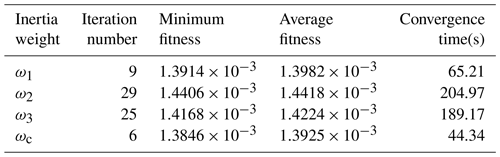The simulation was implemented on a Core (TM) i5-7500 processor with 8 GB of memory. It is obviously found in Table 2 that the COPSO algorithm has a much faster convergence rate and a lower iteration number and is time consuming.

## 4.3 Layered-model inversion

A three-layer and five-layer geoelectric models were investigated, and the PSO parameter values are the same as those of the “Algorithm testing” section in this paper. In order to simulate actual TEM applications, the ramp turn-off is taken into account. Considering the probability distribution characteristic of the above algorithms, the average of 20 simulation results was chosen. The BP, SPSO-BP and COPSO-BP algorithms and a nonlinear programming genetic algorithm (NPGA) (Li et al., 2017) were compared.

### 4.3.1 Three-layer H-type model

The central loop TEM parameters were set as follows: the transmitting coil radius was set to a=100 m; the ramp emission current was set to 100 A; and the turn-off time was set to 1 µs. In the geoelectric model, the resistivity is ρ1=100, ρ2=10 and ρ3=100Ω m and the thickness is h1=100 and h2=200 m.

The BP training samples, which are a series of Hz(t) for different geoelectrical parameters, were generated by the TEM forward model. The resistivity ranges were ρ1(50, 150), ρ2(5, 15) and ρ3(50, 150) and the thickness ranges were h1(50, 150) and h2(100, 300); 1000 random groups were chosen. The resistivity and thickness distributions of ρ1 and h1 were shown in Fig. 6. The relative error is defined as

$\begin{array}{}\text{(21)}& {\mathrm{Err}}_{\mathit{_}\mathrm{rel}}=\left|\frac{{T}_{\mathit{_}\mathrm{cal}}^{\ast }-{O}_{\mathit{_}\mathrm{ref}}^{\ast }}{{O}_{\mathit{_}\mathrm{ref}}^{\ast }}|,\right\end{array}$

where ${T}_{\mathit{_}\mathrm{cal}}^{\ast }$ and ${O}_{\mathit{_}\mathrm{ref}}^{\ast }$ are the calculated and reference values for the geoelectric models.

The inversion results were shown in Table 3 and Figs. 7–8. The BP type algorithms were superior to the NPGA inversion in Table 3. Moreover, the inversion accuracy, convergence rate and optimization ability of the COPSO-BP algorithm were better than others.

Additional results showed that the solution range of ρ1 and h1 in the 20 simulations for the above algorithms were ρ1(97.980, 103.102) and h1(96.962, 102.480) for BP, ρ1(98.954, 101.137) and h1(96.955, 101.829) for SPSO-BP, ρ1(99.382, 100.989) and h1(97.877, 101.044) for COPSO-BP, respectively. Therefore, the COPSO-BP can acquire a higher accuracy and is more stable.

### 4.3.2 Five-layer KHK-type model

A five-layer KHK-type geoelectric model was adopted, and its resistivities were ρ1=100, ρ2=300, ρ3=50, ρ4=200 and ρ5=30Ω m, and its thickness were h1=100, h2=200, h3=300 and h4=500 m.

The training samples with parameter ranges were ρ1(50, 150), ρ2(150,450), ρ3(25, 75), ρ4(100, 300) and ρ5(15, 45) for resistivity and h1(50, 150), h2(100, 300), h3(150, 450) and h4(250, 750) for thickness. The 1000 group training samples were generated within the above ranges. The inversion results were shown in Table 4 and Figs. 9–10. It can be seen that the COPSO-BP algorithm has better global optimization performance.

### 4.3.3 Inversion comparison

Three kinds of BP methods, the traditional BP, SPSO-BP and COPSO-BP algorithms, were compared in Table 5. Hence, the training times of COPSO-BP were obviously less than those of SPSO-BP and were almost equal to BP; it can obtain better precision especially for its global optimization performance.

The inversion of COPSO-BP and NPGA were compared in Fig. 11. The fitting ability of COPSO-BP was much better than NPGA.

Table 6Comparison of inversion results of three-layer H-type (with noise) models.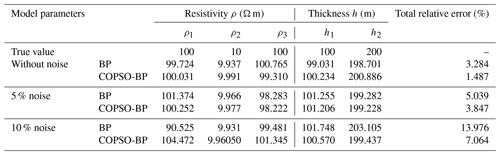### 4.3.4 Robust performance analysis

In order to verify the algorithm robustness, 5 % (26 dB) and 10 % (20 dB) Gaussian random noise was added in TEM data for the three-layer geoelectric model. Three kinds of inversions were implemented respectively. The results and a comparison were shown in Table 6. The Hz(t) and data with 5 % noise were shown in Fig. 12.

As can be seen in Table 2, after applying 5 % and 10 % Gaussian noise, the COPSO-BP inversion has a higher robust ability. The accuracy was obviously improved based on the total relative-error data.

## 4.4 Field example

In order to test the effectiveness of the method, a transient electromagnetic vertical magnetic field (Hz) with 10 measuring points at 380 to 1280 m of the no. 1 line from a mining area in Anhui Province were selected. After the data processing, the inversion was performed using the three-layer neural-network model in the previous section, and the results of the BP and COPSO-BP inversion were compared. Figure 13 shows the comparison between the surveyed data and the inversion data at 380 m of the no. 2 line in the mining area. Figure 14 displays the pseudo-sections of the 10 sets of inversion data combined with the geological data interpolation smoothing. It can be seen from Fig. 14 that the first layer has low resistivity (100–200 Ω m), which is inferred to be the second-layer (T2g22) gray dolomite of the Middle Triassic old Malague section, with a thickness of about 200 m. The second layer has the second-highest resistivity (300–400 Ω m), which is surmised to be the first-layer (T2g21) dolomite of the Middle Triassic old Malague section, with a thickness of about 400 m. The third layer has high resistivity (600–800 Ω m), which is speculated to be the sixth-layer (T2g16) limestone dolomite of the Middle Triassic old group. The results are basically consistent with the geological conditions of the mining area, indicating the feasibility and effectiveness of the neural-network method. And the results of COPSO-BP inversion are better than those of the BP, for which the inversion position is more accurate, the shape and spacing are clearer, and the resistivity of each layer is more consistent with the those of the actual geological model.

5 Discussion

The inversion is performed for three-layer (H-type) and five-layer (KHK-type) geoelectric models in this paper. The results show that the BP neural network is better than the NPGA algorithm because the BP method does not need to use the forward algorithm repeatedly, and its calculation time is short, which is different from the nonlinear heuristic method based on a global space search solution.

The main advantage of the BP is that it can interpret the transient electromagnetic sounding results quickly after training the network. Furthermore, the BP algorithm could automatically obtain the “reasonable rules” between input and output data by learning, and it can adaptively store the learning content in the network weight, for which the BP neural network has a high self-learning and self-adaptation ability. In addition, the superior simulation results of the test function indicate that the BP algorithm can approximate any nonlinear continuous function with arbitrary precision, which means it has a strong nonlinear mapping ability; the inversion results of the layered geoelectric model with uncorrelated noise data prove that the BP algorithm has strong robustness, which means it has the ability to apply learning results to new knowledge. However, the BP neural-network weight is gradually adjusted by the direction of local improvement, which causes the algorithm to fall into a local extremum, and the weight converges to a local minimum that leads to the network training failure. Moreover, the BP is very sensitive to the initial network weight, and the initialization network with different weight values tends to converge at different local minimums, so it obtains different results each time. In addition, the BP algorithm is essentially a gradient descent method, which leads to a slow convergence rate.

From the results of the layered-model and parametric analysis part, it can be seen that the single BP algorithm has a higher error value than SPSO-BP because the BP method is sensitive to initial weight and easy to fall into local minimum values; thus a heuristic global search particle swarm optimization algorithm with a simple structure, rapid convergence and high precision is applied to optimize the weight and threshold of the BP neural network, which improves the global optimization performance of the algorithm. Furthermore, the PSO algorithm adjusts the inertia weight adaptively based on the chaotic-oscillation curve that is similar to the annealing process in the simulated annealing algorithm (SA), which jumps out the local extremum faster in the early stage and accelerates the convergence and reduces the training times. Therefore, compared with the SPSO-BP and BP algorithms, the inversion results of COPSO-BP are closer to the theoretical data with smaller error fluctuations, stronger anti-noise controls, a better generalization of performance and higher stability, which it is effective in solving geophysical inverse problems.

From the simulation experiment, it is not clear how the weight organization affects the BP neural-network weight learning process. It is necessary to conduct a more systematic study on this problem to improve our understanding of how the BP neural network handles training data.

6 Conclusions

The nonlinear COPSO-BP method was proposed for TEM inversion. The BP's initial weight and threshold parameters were trained by the COPSO algorithm, which makes it easy to not fall into a local optimum. The chaotic-oscillation inertia weight for PSO was proposed so as to improve the PSO's global optimization ability and fast convergence in the early stage. The layered geoelectric model inversion showed that the COPSO-BP method is more accurate and stable and requires relatively less training time.

Code availability

The PSOBP code was developed by Huaiqing Zhang and Ruiyou Li of Chongqing University in China, who are able to be contacted at the telephone number 13752954568 or e-mail address zhanghuaiqing@cqu.edu.cn. A computer with MATLAB R2016a is required is to run this code. The programming language of this code is C$++$, and its size is 10 KB. The source code is available at https://github.com/liruiyou/PSOBP (last access: 22 February 2019).

Author contributions

HZ conceived this paper. RL and HZ developed the main algorithmic idea and the mathematical part. RL and NY carried out the simulation and data analysis. QZ completed the writing and interpretation of this paper. All authors contributed to the writing of the paper and approved the final paper.

Competing interests

The authors declare that they have no conflict of interest.

Acknowledgements

This work was partly supported by the National Natural Science Foundation of China (grant no. 51377174).

Financial support

This research has been supported by the National Natural Science Foundation of China (grant no. 51377174).

Review statement

This paper was edited by Norbert Marwan and reviewed by two anonymous referees.

References

Dai, Q., Jiang, F., and Dong, L.: Nonlinear inversion for electrical resistivity tomography based on chaotic DE-BP algorithm, J. Cent. South. Univ., 21, 2018–2025, https://doi.org/10.1007/s11771-014-2151-9, 2014.

Fernández Martínez, J. L., García Gonzalo, E., Fernández Álvarez, J. P., Kuzma, H. A., and Menéndez Pérez, C. O.: PSO: A powerful algorithm to solve geophysical inverse problems: Application to a 1D-DC resistivity case, J. Appl. Geophys., 71, 13–25, https://doi.org/10.1016/j.jappgeo.2010.02.001, 2010.

Godio, A. and Santilano, A.: On the optimization of electromagnetic geophysical data: Application of the PSO algorithm, J. Appl. Geophys., 148, 163–174, https://doi.org/10.1016/j.jappgeo.2017.11.016, 2018.

Jha, M. K., Kumar, S., and Chowdhury, A.: Vertical electrical sounding survey and resistivity inversion using genetic algorithm optimization technique, J. Hydrol., 359, 71–87, https://doi.org/10.1016/j.jhydrol.2008.06.018, 2008.

Jiang, F., Dai, Q., and Dong, L.: An ICPSO-RBFNN nonlinear inversion for electrical resistivity imaging, J. Cent. South. Univ., 23, 2129–2138, https://doi.org/10.1007/s11771-016-3269-8, 2016a.

Jiang, F., Dai, Q., and Dong, L.: Nonlinear inversion of electrical resistivity imaging using pruning Bayesian neural networks, J. Appl. Geophys., 13, 267–278, https://doi.org/10.1007/s11770-016-0561-1, 2016b.

Jiang, F., Dong, L., and Dai, Q.: Electrical resistivity imaging inversion: An ISFLA trained kernel principal component wavelet neural network approach, Neural. Networks., 104, 114–123, https://doi.org/10.1016/j.neunet.2018.04.012, 2018.

Johnson, O. L. and Aizebeokhai, A. P.: Application of Artificial Neural Network for the Inversion of Electrical Resistivity Data, Journal of Informatics and Mathematical Sciences, 9, 297–316, 2017.

Kaufman, A. A. and Keller, G. V.: Frequency and Transient Sounding, Elsevier Methods in Geochemistry & Geophysics No 16, 620 pp., 1983.

Li, F. P., Yang, H. Y., and Liu, X. H.: Nonlinear programming genetic algorithm in transient electromagnetic inversion, Geophysical and Geochemical Exploration, 41, 347–353, 2017.

Li, Y. Y., Chen, B. C., Zhao, Y. G., Yun, C., Ma, X. B., and Kong, X. R.: Nonlinear inversion for electrical resistivity tomography, Chinese J. Geophys., 52, 758–764, https://doi.org/10.1016/S1003-6326(09)60084-4, 2009.

Maiti, S., Erram, V. C., Gupta, G., and Tiwari, R. K.: ANN based inversion of DC resistivity data for groundwater exploration in hard rock terrain of western Maharashtra (India), J. Hydrol., 464, 294–308, https://doi.org/10.1016/j.jhydrol.2012.07.020, 2012.

Pekşen, E., Yas, T., and Kıyak, A.: 1-D DC Resistivity Modeling and Interpretation in Anisotropic Media Using Particle Swarm Optimization, Pure Appl. Geophys., 171, 2371–2389, https://doi.org/10.1007/s00024-014-0802-2, 2014.

Raj, A. S., Srinivas, Y., and Oliver, D. H.: A novel and generalized approach in the inversion of geoelectrical resistivity data using Artificial Neural Networks (ANN), J. Earth Syst. Sc., 123, 395–411, https://doi.org/10.1007/s12040-014-0402-7, 2014.

Rosas-Carbajal, M., Linde, N., Kalscheuer, T., and Vrugt, J. A.: Two-dimensional probabilistic inversion of plane-wave electromagnetic data: methodology, model constraints and joint inversion with electrical resistivity data, Geophys. J. Int., 196, 1508–1524, https://doi.org/10.1093/gji/ggt482, 2014.

Sharma, S. P.: VFSARES – a very fast simulated annealing FORTRAN program for interpretation of 1-D DC resistivity sounding data from various electrode arrays, Comput. Geosci., 42, 177–188, https://doi.org/10.1016/j.cageo.2011.08.029, 2012.

Shi, F., Wang, X. C., and Yun, L.: Matlab neural network case study, The Beijing University of Aeronautics & Astronautics Press, Beijing, 2010.

Shi, X. M., Xiao, M., Fan, J. K., Yang, G. S., and Zhang, X. H.: The damped PSO algorithm and its application for magnetotelluric sounding data inversion, Chinese J. Geophys., 52, 1114–1120, https://doi.org/10.3969/j.issn.0001-5733.2009.04.029, 2009.

Srinivas, Y., Raj, A. S., Oliver, D. H., Muthuraj, D., and Chandrasekar, N.: A robust behavior of Feed Forward Back propagation algorithm of Artificial Neural Networks in the application of vertical electrical sounding data inversion, Geosci. Front., 3, 729–736, https://doi.org/10.1016/j.gsf.2012.02.003, 2012.

Tran, K. T. and Hiltunen, D. R.: Two-Dimensional Inversion of Full Waveforms Using Simulated Annealing, J. Geotech. Geoenviron. Eng., 138, 1075–1090, https://doi.org/10.1061/(ASCE)GT.1943-5606.0000685, 2012.

Wang, H., Liu M. L., Xi, Z. Z., Peng, X. L., and He, H.: Magnetotelluric inversion based on BP neural network optimized by genetic algorithm, Chinese J. Geophys., 61, 1563–1575 https://doi.org/10.6038/cjg2018L0064, 2018.

Zhang, L. Y. and Liu, H. F.: The application of ABP method in high-density resistivity method inversion, Chinese J. Geophys., 54, 64–71, https://doi.org/10.1002/cjg2.1587, 2011.